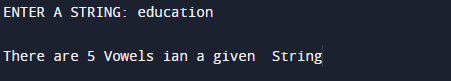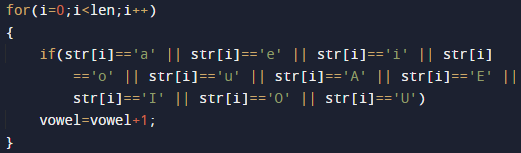C Program To Count number of vowels in a string

In this tutorial you will learn about the C Program To Count number of vowels in a string and its application with practical example.

In this tutorial, we will learn to create a C program that  will find the number of vowels in a string  using C programming.

Prerequisites

Before starting with this tutorial we assume that you are best aware of the following C programming topics:

• Basic input/output.
• loop statements.
• for Loop.
• Array.

What Is  vowel ?

Let us take an example, in the following string “Education” there are five vowels ‘a’,’e’,’i’,’o’ and ‘u’. here in this program we check every character inputted by user in a string and find the number of vowel in it.

C Program To Count number of vowels in a string.

First of all In this program we take string from user,and with the help of array and loop we will find number of vowel in given string.

OutputIn the above program, we have first declared and initialized a set variables required in the program.

• str = number of terms to be printed
• len = counting length of string
• vowel=  for counting number of vowel
• i= for iteration of loop.

Explanation.

In this program we will do following task step by step.

• First of all we will take string as input.
• then with help of strlen() function we find the length of a string.• Then check  each character of the string to check as shown in above  image.
• If the string  character is a vowel, increment the counter value of vowels.
• Print the total number of vowels in the end.
In this tutorial we have learn about the C Program To Count number of vowels in a string and its application with practical example. I hope you will like this tutorial.